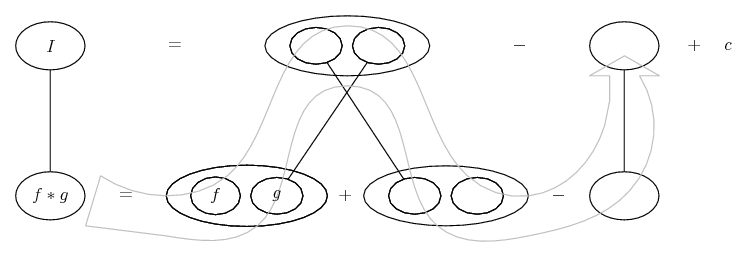# Thread: Integration By Parts

1. ## Integration By Parts

I am doing integration by parts and am struggling a little bit with two revision questions:

The first is:

1) \int cos(2x).(5x+1).dx

The answer I have is - (5x+1)sin2x/2+5/4cos2x

Is this right although I am not sure how I got there.

The other question is a Definite Integral:

2) \int (x+2)sinx.dx and the limits are 1 and 2

I just can't seem to get the right indefinite integral to be able to replace x with the limits.

The answer I get is (x+2)(-cos(x))+sin(x) but when O replace x with the upper and lower limit I get the wrong answer.

Can anyone please help me.

Thanks

M

2. 1) is correct. Will post a pic to show how.

2) Are you subtracting cos x, there, or... should there be brackets...?

1) Just in case a picture helps...... where (key in spoiler) ...

Spoiler:... is the product rule. Straight continuous lines differentiate downwards (integrate up) with respect to x. And,... is lazy integration by parts, doing without u and v.

2) is much the same... you try._________________________________________

Don't integrate - balloontegrate!

Balloon Calculus; standard integrals, derivatives and methods

Balloon Calculus Drawing with LaTeX and Asymptote!

3. OK, you've now put the brackets in... is that not working?

Again, just in case...(And follow the key in post 2.)

_________________________________________

Don't integrate - balloontegrate!

Balloon Calculus; standard integrals, derivatives and methods

Balloon Calculus Drawing with LaTeX and Asymptote!

4. Thank you that makes sense. Are you also able to help me with the following as well?

∫(7-x)sin(x).dx

I know the answer is:

(x-7).cos(x)-sin(x)

but i can't figure out why the (7-x) is swapped over and becomes (x-7).

M

5. (x - 7) = - (7 - x)

The negative belonged originally to the cos (but then to the whole product). I.e. it's just a neat way of removing the negative.

We could balloonify it as either...... or..._________________________________________

Don't integrate - balloontegrate!

Balloon Calculus; standard integrals, derivatives and methods

Balloon Calculus Drawing with LaTeX and Asymptote!

#### Search Tags

integration, parts